# Order from Order: 3D Fractals from Quaternions and Hypercomplex Numbers

Fractals from Quaternions as wholes

3D Fractals more generally (even with no cylindrical symmetry) can be obtained thanks to generalizations of the usual complex numbers like, e.g., Quaternions or Hypercomplex numbers. In both cases the number of degrees of freedom are raised from 2 (1 real and 1 imaginary part of usual complex numbers) up to 4 (1 real and 3 imaginary parts of quaternions or hypercomplex numbers). Since only 3 co-ordinates can be represented in a plot, the image resulting will be a projection onto the usual 3D space of a 4D fractal structure.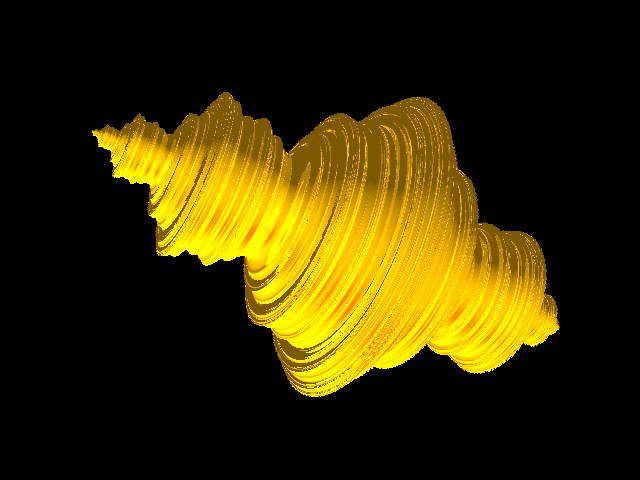Julia 3d (C =-0.7454294)

POV Ray 3.7 code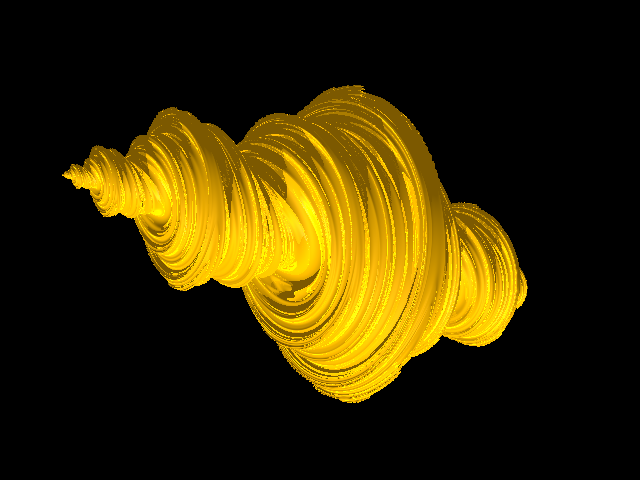Julia 3d (C =-0.7454294 + j 0.25)

POV Ray 3.7 code

Fractals from Hypercomplex Numbers as wholesJulia 3d (C =-0.7454294)

POV Ray 3.7 code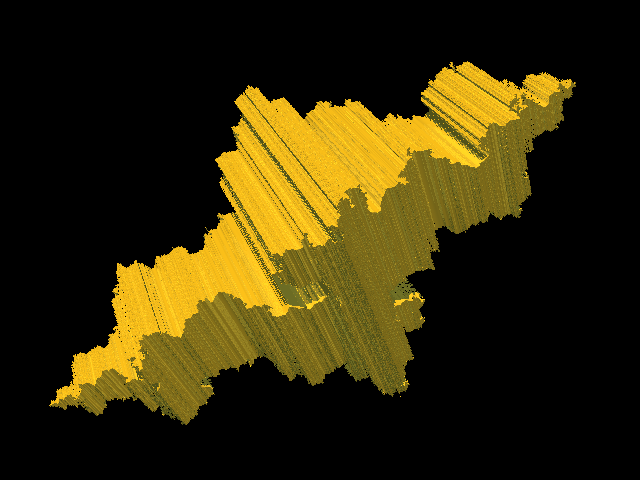Julia 3d (C =-0.7454294 + i o.113089 +

+ j 0.113089)

POV Ray 3.7 code

Sequentially ordered "point by point" generation allows to combine Mandelbrot or Julia like recurring law according to several different combinations so obtaining e.g. either Mandelbrot-Mandelbrot-Mandelbrot, or Julia-Julia-Julia, or Julia-Mandelbrot-Mandelbrot, etc. structures.

Fractals from Quaternions plotted sequentially

Mandelbrot-Mandelbrot-Mandelbrotsection 1section 2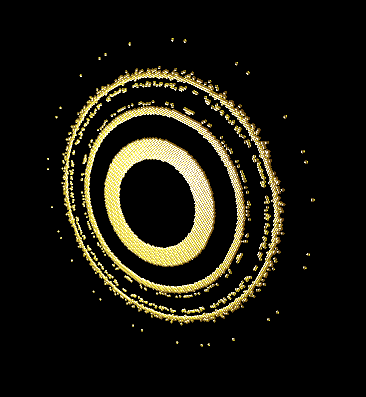section 3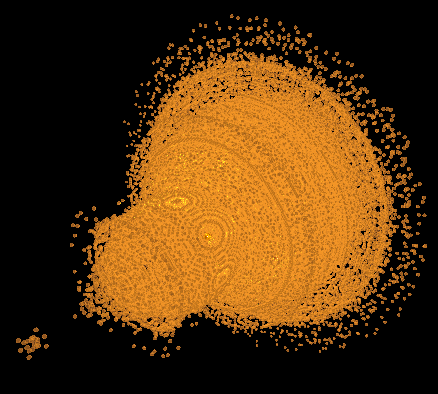POV Ray 3.7 code

view animation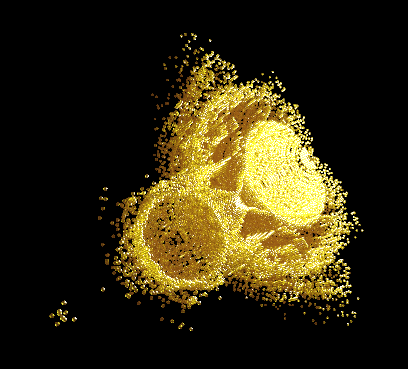open section

Julia-Julia-Julia (C = -0.7454294)section 1section 2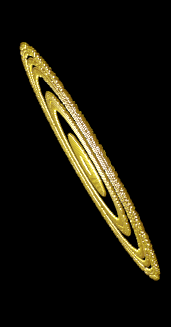section 3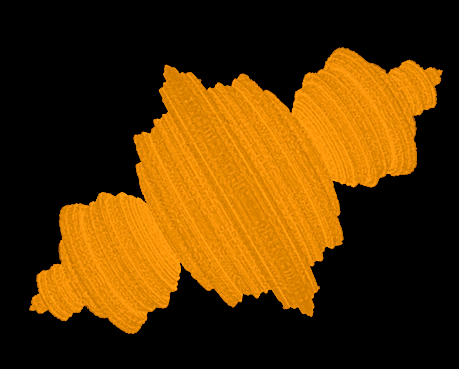POV Ray 3.7 code

view animation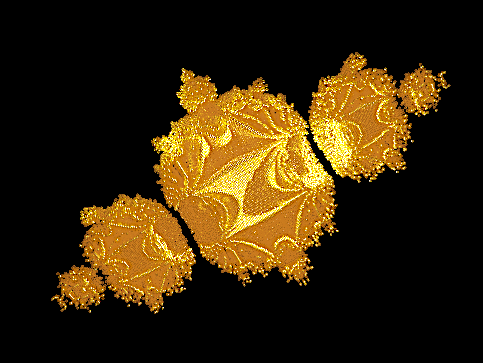open section

Julia-Mandelbrot-Mandelbrot (C = -0.7454294)section 1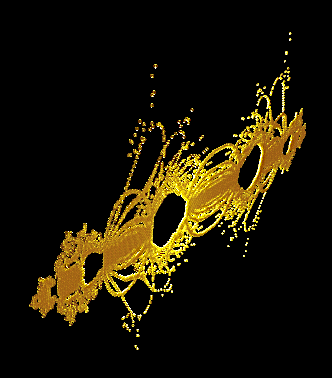section 2section 3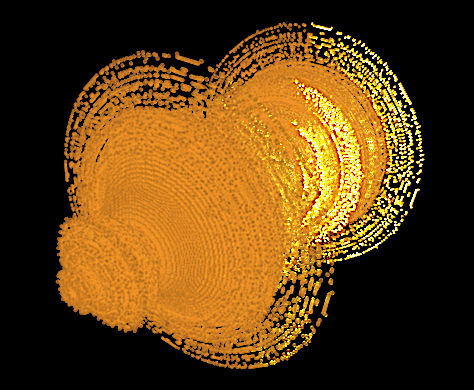POV Ray 3.7 code

view animation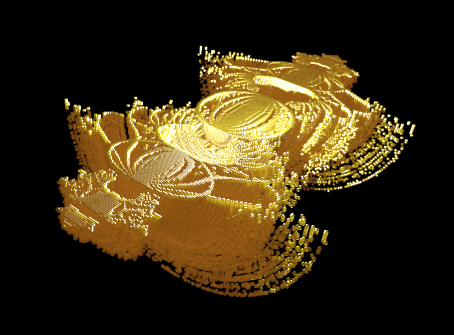open section